# Introduction to STL in C++

## Introduction to STL in C++:

In this article, I am going to give a brief Introduction to STL (Standard Template Library) in C++. Please read our previous article where we discussed File Handling in C++ with Examples. At the end of this article, you will understand what are STL and why we need STL in the C++ language.

##### What is STL in C++?

STL stands for Standard Template Library. It is the set of tools available in C++ programming platforms. So whatever platform we are using these STL will be there. Let us first understand why we need STL. We have written so many programs. We have declared simple variables, and we have defined our classes and created objects for those classes. We have written functions and passed the parameters. So, all the programs that we have written till now, are simple programs for learning the features of C++.

##### Why do we need STL in C++?

STL in C++ helps us to code quickly, efficiently, and in a generic way. So why quickly? Because most of the data structures and algorithms that we need to use in our code are already implemented in our library.

For example, you want to design an application where you need some sort of priority queue. So instead of designing a priority queue by yourself, you can directly use the implementation of a priority queue in C++ STL. So, it will help you to write your code much more quickly. Similarly, these implementations have been there for a long time and these have evolved over time. These have stood the test of time and they are very efficient. And in the recent implementation, even some of the abstractions are being optimized. So that you do not get any overhead due to different abstraction levels.

So, these are very efficient implementations of the data structures and algorithms. Also, these are generic programming paradigms since the implementations do not take into account a particular type. But they are type agnostic and when you are using it, you have to provide the type. So, a simple way would be that let us say you have a stack. It does not matter whether you are inserting an int into the stack or a string or a custom class into the stack, the stack will always be implemented in the same way that it has to follow last in first out. Whatever element is pushed last, when we pop then the last element will be popped first.

So, these follow a generic programming paradigm. Let us see what is generic programming paradigm and then we will see how C++ handles it.

##### Generic Programming in C++:

Generic programming is a programming paradigm where data types are not specified in the implementation of the code. But rather they are specified while using those implementations. Let us take the same example of the stack. For stack, we do not need to know the type of data being inserted in the stack for implementing the stack. So, here, most of the implementations are generic. That is why the name is the template.

Sometimes these are also called compile-time polymorphism. Because specifying data types at variable definition or object instantiation is in essence polymorphism which is resolved at compile time. So, this is potentially more efficient than runtime polymorphism where some CPU cycles are consumed. But here it is all done at compile time so these are much more efficient. Now let’s see how C++ achieves this generic programming.

##### Generic Types in C++: Templates

In C++, there are generic types which are called templates. You can see the keyword Template which is present in the standard template library (STL). The Templates provide generic programming in C++ and these are special constructs. So, if we want to define a function that calculates the area of a rectangle then we can do it as,

int area(int a, int b){
int result = a * b;
return result;
}

But let’s say we want to implement an area for float and double also. So, the above code will not work or even if you provide input i.e. 10 and 5.5 then 5.5 will be converted into int and then the area will be calculated. So, our calculation will go wrong. So, the generic way of doing this would be to use templates. So, we will create the same function as,

template <typename T>
T area(T a, T b){
T result = a * b;
return result;
}

Here, first, we have written the keyword “template” then inside angular braces, we have written typename or you can use the class keyword here. Generally, we use class for defining generic classes. You can have template functions as well as classes. STL library contains both functions as well as classes. We can write the typename of the class and after this, we have to write T or any name instead of T.

Here we have just used one type but you can assign multiple i.e. T1, T2, and so on. Here, T is a generic type and our implementation is based on this generic type. So, the return type is T and it takes two parameters of type T. And then we have the result of type T then we have returned the result. It should be the area of a rectangle rather than a simple area. And we will call this generic function as,

int x = area<int>(4, 8);
double y = area<double>(3.3, 1.5);
float z = area<float>(2.3f, 1.2f);

Here, at the time of implementation of the area, we are passing the type in the angular braces. This is a very simple example but we have very complex functions and algorithms that we are implementing in this generic way. It is very useful and it can be used with different types. Now let us see the different components of STL.

##### Components of STL in C++:

There are four components of STL:

1. Containers
2. Iterators
3. Algorithms
4. Functors (Function Objects)

Containers are just like array data structures that store a collection of objects. You need some data structure where you store it. Then we have iterators. These are like pointers objects that allow the traversal of containers and we will see different types of iterators in coming articles. We will also look at different containers. Then we have algorithms. These are a set of functions that are implemented in an efficient way and these implement different algorithm operations such as search, sort, modify, count, etc. There are many more algorithms and these are generally defined in algorithm.h. You need to include <algorithm>.

Then we have functors or function objects. These are classes that override and overload the parenthesis operator such that they can be used as functions that maintain state and can be parameterized and these are generally defined in <functional.h>. Some examples of functional.h are std::plus, equal to, and so on. These are generally used with other functions. Std::plus is a binary operator so this is defined for two parameters. There are some unary things also. We will see different components in the upcoming articles.

##### Example to Understand Generic Types in C++:
```#include <iostream>
using namespace std;

template <typename T>
T area(T a, T b){
T result = a * b;
return result;
}

int main()
{
int x = area<int>(4, 8);
double y = area<double>(3.3, 1.5);
float z = area<float>(2.3f, 1.2f);

cout << "x: " << x << " y: " << y << " z: " << z;

return 0;
}
```
###### Output: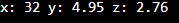When you develop any application then you have to deal with the data. So, what will be the size of the data? This is a very important thing. So, when your program or application will be dealing with one or two values or the list of the values, then where you will store the collection of the values? So, for storing the collection of values, we need Data Structures.

Data Structure is one of the important topics in computer science. This is a subject that has various types of data structures. In academics, engineering students study this subject and tried to write the programs by themselves for implementing data structures.

##### What is Data Structure?

It is a collection of data and the arrangement of the data for its efficient utilization. So, depending upon your utilization, you can arrange the data so that it can be utilized efficiently. Efficiency in terms of time and space. So, we want the data to be stored and retrieved easily and also occupy less space. When you have a collection of data so where do you store that data? Inside the data structure.

##### What Data Structures are available in C++?

Let us first take the example of data. Which type of data do we want to deal with? So, let’s say we want to deal with the marks of students in a particular subject. We have marks of 5 students and upon that we want to find which is maximum or minimum. So, we want to perform so many operations like sorting those marks, finding the average, etc.

Let us take an example of 5 students’ marks. So where do you keep those marks? We keep them in an array. We create an empty array of size 5 as shown in the below image.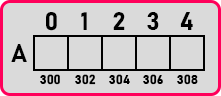In the above empty array, we store the marks of 5 students as follows.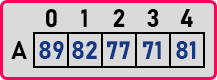We have filled the marks of 5 students. This is an array. The built-in data structure available in C++ or mostly in any other programming language is an Array. An array is a collection of similar types of elements. Marks are integers, so we have to declare an array like

int A;
int *A = new int;

Above are the two methods of declaring an array of size 5. The First method will create the array inside the stack and the second method will create the array inside the heap which is dynamic memory allocation.

Now, what can we do with these 5 numbers which are stored in the array? We can display all the numbers, we can find the sum of all the numbers, can find the average, the maximum, and the minimum of these numbers, and we can also apply some arithmetic conditions to these numbers like how many students got marks more than 50 or 80, etc. There are lots of things we can do on this array.

So, when you have some collection of data you can do a lot of analytical work or perform lots of operations. We have taken an example of only numbers. You can take any application. Like, suppose a reminder app on your mobile phone. It will have a list of entries with the date and time and the message you want. Like this, there is a list of entries. So, it will be an array of entries. So, whenever you open the remainder app, it has to load all the entries from the memory and keep them in an array.

Let us take the example of the music player on your mobile phone. When you open the music player it will find out all the songs which are available on your phone and then show the name of all those songs. The app has to bring all the songs into an array and then show them. Like these, there are many examples available where an array is used for storing the collection of values.

Can we replace any number in the array? Yes, we can replace it like in the array. In the array, A has a value of 77. We can change this number to any other number like,

A = 75;

So now the value of A is changed to 75 as shown in the below image.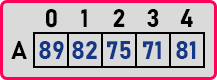Now can we insert some numbers into this array? Suppose, we want to insert 92, so can we insert it at the end of the array? But there is no space in the array. That’s the point. Whenever you create an array, you must be sure about the size of the array you want. Because once the array is created then the array size cannot be changed. It cannot be increased or decreased. So, this is the problem with the array. The arrays are available in C++ by default. But the problem is that their size is fixed. So initially you should know the size.

Suppose the size of the array is 100 but we are storing only 10 numbers. So, the problem here is that the probability of utilizing an array with exact space is less. Either it may find insufficient space or we can face the problem that a lot of space is wasted in the array. So, this is a common problem found in the built-in data structure of C++. Adding more values or deleting the values, are the common operations on the data structure. So, in the array, the problem is space. Let us see how this problem can be solved. Please have a look at the following array. Here, we have created an array of size 10.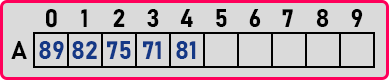In the above array, suppose we want to insert 84 at index 3 then what should we do? Move all the elements from index 3 to right free spaces then we can insert 84 at index 3. Suppose we want to delete 75 then we have to move all the right-hand elements by 1 towards the left side. So that the data in the array will remain contiguous. So, for deletion and insertion, data movement is required.

Now let us take a situation where we are expecting not more than 10 numbers. In our program, we have created an array of sizes 10. And then we have given our program to the client or user. Then the client has entered more than 10 numbers then how my program can manage it? My program will crash and then the client will complain to me that he/she cannot store more than 10 numbers in the program. We should make our program smart enough so that even if the client enters more than 10 numbers then my program should store all the numbers. So how it is possible? The array cannot be updated. Let us see the one logic to increase the size of the array.

See we cannot increase the size of an array but we can create a new array with a bigger size. Then we can copy all the elements from the older array to the new array. Suppose we have an array of size 5 which is already full then we can create another array of size 10 and copy all those elements into the new array as shown in the below image.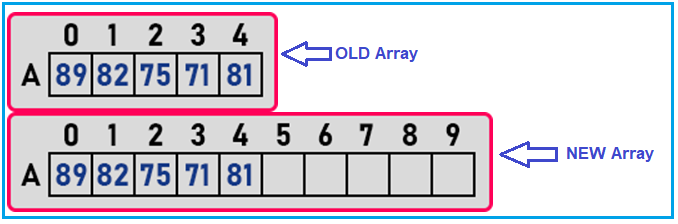This is possible if you are creating the array dynamically. The array A was pointing to the 5 blocks of memory then we have pointed A to 10 blocks of memory like,

int A = new int ;
A = new int ;

So, I have given you the idea to increase the size of an array. This is the common logic used by any programmer.

##### Types of Data Structures:

We have already seen one data structure which is an array. And we have discussed the problem with arrays which is their size that cannot be increased or decreased. Then we have also seen the solution to increase or decrease the array size.

##### Is there any other data structure available?

Yes, there is one more data structure available which is Linked List.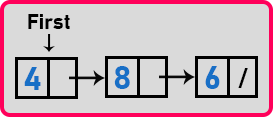Instead of having a fixed-size array, we can have a collection of nodes where each node can have the values. Like, in the above-linked list, each node has some value i.e. 4, 8, 6, etc.

The benefit of a linked list is that the size of a linked list can grow or reduce when the numbers are inserted or deleted. This will take an amount of space depending on the number. So, the size is variable. You don’t have to create a bigger size and transfer anything that we have discussed in the array data structure. Simply we can add the nodes and delete the nodes. Suppose in the above-linked list we want to add 7 at the end, then we can add as follows.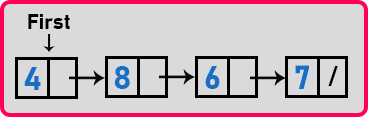So, we can insert it easily at any position in the linked list. Here 4 is the head node and 7 is the last node. So, this is a singly linked list. Here every node has a single pointer that is forward direction only. Here you can traverse the elements in the forward direction only. And there is a doubly linked list also. For a better understanding, please have a look at the following image. The following diagram shows a doubly linked list.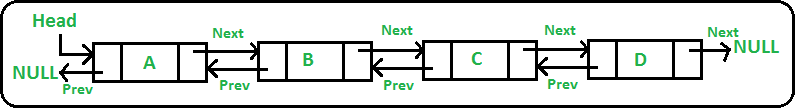This is having two pointers that are for pointing next node as well as the previous node. Here you can traverse the elements either in a forward or backward direction.

These are the data structures. Mostly, students implement them by writing the programs like data structures using C or C++. They write the program for the array or linked list. And among these, how do you utilize data structure? How do you insert and delete the values? Depending on that, there are some more data structures that are

1. Stack
2. Queue
3. Deque
4. Priority Queue
5. Map
6. Set

These are the data structures commonly used. And these are the data structures used for developing applications. We use stack and queue to store the elements.

Now, as a programmer do I have to write down the code for the linked list and array, or should I completely write the complete program for implementing Stack or Queue? No. C++ provides a built-in library of classes for all these things. And that is the collection of classes called STL in C++. So, there is a collection of header files, that contains many classes in them, and that collection is called STL. So, for every data structure, there is a class available. And in our upcoming articles, we will discuss all those data structures or STL Classes in detail with Examples.

In the next article, I am going to discuss STL Container Classes in C++ with Examples. Here, in this article, we have discussed what is data structure, the need for the data structure that we have to store the collection of values, then the built-in data structure that is an array, look at the size problem, and see the available solution. We have also discussed Linked List Data Structure and given an introduction to STL in C++ and I hope you enjoy this brief Introduction to STL in C++ article.